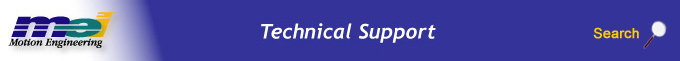.

# Determining Required Compensator Table Size

The compensator table size is dependent on the number of dimensions (1D or 2D), the position range, and the resolution (or granularity) of the compensation points. The compensator uses linear interpolation to calculate the compensation values between each distinct compensation point.

For each compensation axis there are three position values: Min, Max, and Delta. The compensating range for an axis is specified by the Min and Max positions along the axis. The range (Max – Min) divided by Delta, determines the number of required points for the compensator. You can calculate the number of required compensator points by using the following equations:

1D Compensation: Points = (positionMax-positionMin) / positionDelta + 1

2D Compensation: Points = PointsX * PointsY

NOTE: Delta must be an exact multiple of the difference between Min and Max.

Example: (taken from comp.c sample application)

To compensate a Z (vertical) axis for X-Y surface irregularities, first define the X-Y area to be compensated (Xmin to Xmax, Ymin to Ymax). Then define the spacing of the measuring points (delta) for the X and Y axes to determine the compensation table size.

For the X-Y table diagram below we have:
Xmin = 0, Xmax = 200000, Xdelta = 50000
Ymin = 25000, Ymax = 225000, Ydelta = 10000

###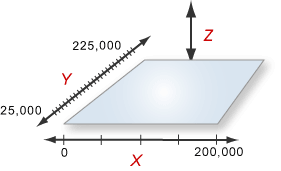For this table our X & Y dimensions are:
X_DIM = (200000-0)/50000 + 1 = 5
Y_DIM = (225000-25000)/10000 +1 = 21

which requires a table point count of:
Points = X_DIM * Y_DIM = 105

With this information we can now configure the size of our compensation table on the controller using MPIControlConfig.compensatorPointCount = 105.

Because the compensator table data is stored directly on the controller, it is important to know how much memory is available. This information can be found using Motion Console under the Controller Summary Window > Config Tab.

The three screenshots below show the available memory for no compensator table, compensator table with 100 points, and compensator table with 1000 points, respectively. Having 100 points requires 400 (156272 – 156672) bytes of controller memory and having 1000 points requires 4000 (156672 – 152672).

 No Compensator Table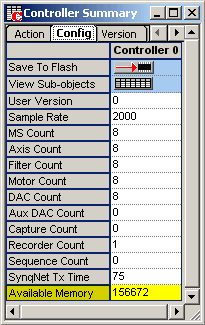Compensator Table (size = 100)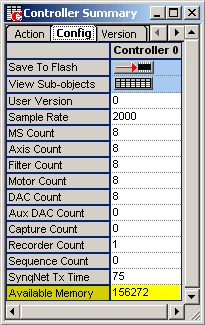Compensator Table (size = 1000)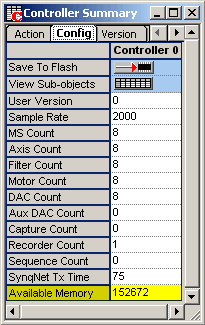Each data point will require 4 bytes of controller memory. In the "No Compenstor Table" example above, a maximum of 39168 (156672 / 4) points can be created.

NOTE: Although having more compensator points gives a better representation of a system; it would also significantly increase the time needed to gather all of the required data. For instance, if you added 20000 points and it took an average of 5 seconds to collect the data for that point, it would take you 100000 (20000 * 5) seconds = 1.16 days to collect that data.

 Legal Notice  |  Tech Email  |  Feedback        Copyright © 2001-2010 Motion Engineering